# Effect of Heat Transfer on Newtonian Flow Between Concentric Elliptical Regions

Effect of Heat Transfer on Newtonian Flow Between Concentric Elliptical Regions
Department of Mathematics, Nitte Meenakshi institute of Technology, Bengaluru, Karnataka 560064, India

Department of Mathematics, School of Applied Sciences, REVA University, Bengaluru, Karnataka 560064, India

Corresponding Author Email:
sushma.puranik28@gmail.com
Page:
1015-1025
|
DOI:
https://doi.org/10.18280/ijht.390339
20 August 2020
|
Accepted:
25 May 2021
|
Published:
30 June 2021
| Citation

OPEN ACCESS

Abstract:

In the present study, the slow and steady flow of Newtonian fluid in the presence of heat transfer in annular region formed by two concentric ellipses is considered. The concentric ellipses in z-plane are mapped to concentric circles in ζ-plane using conformal transformation. Analytical solutions for velocity and temperature are obtained in  ζ-plane and the same are graphically depicted. The effects of dimensionless parameter such as Grashof number Gr, heat flux β, initial temperature at wall of inner ellipse θ0 and area of cross section of concentric ellipses on velocity, rate of flow and temperature have been studied. The graphical representation shows that the velocity, temperature is enhanced with increase in Gr and area of cross section between ellipses, and decreases with increase in heat flux β.

Keywords:

conformal mapping, concentric ellipse, doubly connected region, heat transfer, Newtonian fluid

1. Introduction

Numerous industrial applications, where heat load is considerable and space is constrained, require the utilization of tubular heat exchangers for cooling of electronic hardware. It is found that elliptical geometries perform better than circular geometries in these applications . The Newtonian and non-Newtonian fluids flow in the presence of heat transfer, across circular and non-circular cross-sectional region includes many applications. Some of them include flow in, tubular and hair pin heat exchangers, hot wire anemometer, screen filters and aerosol filters, resin transfer molding process of manufacturing of fiber- reinforced composites . The classical example of this flow problem is used in transport phenomenon.

There have been extensive studies on fluid flow and heat transfer in doubly connected region. Shivakumar and Ji  discussed the solution of Poisson’s equation for a doubly connected region formed by two curves C1 and C2. They have compared rate of flow in case of concentric circles and eccentric circles. Flow through an annular region bounded by non-concentric ellipses or by an ellipse in a circle has been analyzed by Williams et al. . They have calculated the axial and cross-sectional resistance numerically, to determine their dependence on cross-sectional geometry which includes, a circle in an ellipse, an ellipse in a circle and ellipse in an ellipse of equal eccentricity and orientation. Saatdijan et al.  studied analytical solution of Stokes equation between confocal ellipses. Here the flow in annular region between two concentric ellipses is studied such that the boundaries are allowed to slide elliptically in both clockwise and anticlockwise direction. The Von Karman - Pohlhausen integral method is used by Khan et al.  to solve average heat transfer from energy equation and total drug from momentum equation in flow across an elliptical cylinder under isothermal boundary conditions. It is presented in this paper that elliptic cylinders provide less resistance to flow and better insulation for heat transfer compared to circular cylinders. J.P.B. Mota et al.  developed efficient code to solve 2D Darcy- Boussinessq equations, governing Natural convection heat transfer in horizontal eccentric elliptic annular region containing saturated porous media. D’alessio and Dennis  have devised numerical technique to solve two-dimensional steady state problem of laminar forced convection in isothermal cylinder whose cross section is elliptic. In this elliptic region, the asymptotic behavior of Navier-Stokes equation and energy equation is studied by conformal mapping it to semi-infinite rectangular strip. Bharti et al.  studied flow of power law fluid past the heated elliptical cylinders through forced convection. Saatdijian et al.  investigated experimental and numerical results of chaotic mixing and heat transfer in confocal ellipses. From experimental results they have analyzed that better mixing is obtained when the inner ellipse displacement per period increases and confocal ellipses shown to be particularly effective as a heat exchanger. Indira et al.  have considered analytical study of pulsatile flow of couple stress fluid in doubly connected region. Shivakumar  considered viscous flow in pipes whose cross section are bounded by an ellipse on the outside and circle on inside. The outer ellipse in z-plane is conformal mapped to circle in ζ-plane.

As seen above, there have been many works on Newtonian fluid inside or past the circular or elliptical geometries. There have been certain works where the flow is considered in a doubly connected region [3-5, 9-13]. These regions comprises of circle inside ellipse [4, 10], square inside circle , eccentric circles [3, 4, 9]. These works are restricted to only on flow of fluid. Some of the works are only on flow of different type of fluids either inside elliptic region or past the elliptic cylinders [5, 11, 14]. The introduction to heat transfer is done in , whose cross section is eccentric ellipses. But this work is on saturated porous media between eccentric annuli of ellipses. Also, few studies are available on heat transfer past or across the elliptic cylinders [1, 2, 7, 14]. Shivakumar  has studied viscous flow between ellipse and circle, and investigated the rate of flow on three geometries which includes concentric circles, concentric ellipses, a circle inside ellipse and made comparison between them. Studying above all works, the present study is extended to heat transfer in fluid flow between concentric ellipses. The method of solution used in study is followed from Shivakumar  and results of rate of flow in present study can be compared to Shivakumar . The advantage of using conformal mapping technique are, resulting partial derivatives obtained from governing equations can be solved easily and closed form of solution is obtained.

The present work deals with study of effect of heat transfer on fully developed, steady state, Newtonian flow between two concentric ellipses. The boundary conditions are applied by mapping concentric ellipses to concentric circles using appropriate conformal transformation. This study is restricted to low Reynold’s number and thus the velocity, temperature of fluid, rate of flow are studied for following ranges of Grashof number 1<Gr<10, initial temperature 0.1<θ0<1, heat flux β=0.001, 0.01 and area of cross section ε=0.28, 0.31, 0.40.

2. Mathematical Formulation

In the present study Newtonian, steady, incompressible, fully-developed, viscous flow with low Reynold’s number between two rigid, impermeable long concentric elliptic tubes, whose cross section is concentric ellipses are considered. The inner ellipse is assumed to be at temperature θ=θ0, while outer ellipse is at temperature θ=0 and heat is transferred through flow from inner ellipse to outer ellipse due to free convection. The effect of heat transfer on flow between two concentric ellipses is studied. The physical configuration of problem is given in Figure 1 and cross section of concentric elliptical tubes is shown in Figures 2a, 2b and 2c, accordingly with different area of cross section.

## 1.jpg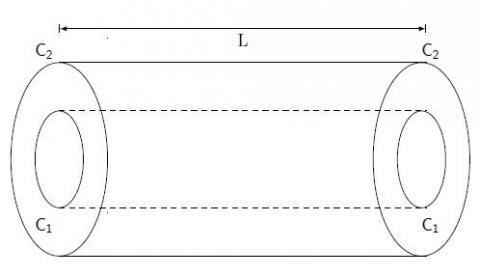Figure 1. Physical configuration of problem

## 2a.png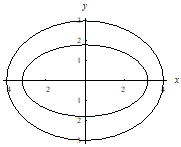(a) α1=3.2, β1=1.8, α2=4, β2=3, ε=0.28

## 2b.png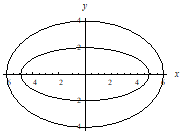(b) α1=4.9, β1=2, α2=6, β2=4, ε=0.31

## 2c.png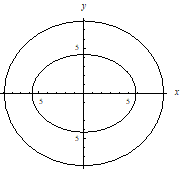(c) α1=5.8, β1=4.3, α2=8.9, β2=8, ε=0.40

Figure 2. Cross section of concentric elliptic tubes

Two concentric, rigid, impermeable ellipses are given by,

$C_{1}: \frac{x^{2}}{\alpha_{1}^{2}}+\frac{y^{2}}{\beta_{1}^{2}}=1, \quad \alpha_{1}>\beta_{1}, \quad C_{2}: \frac{x^{2}}{\alpha_{2}^{2}}+\frac{y^{2}}{\beta_{2}^{2}}=1, \quad \alpha_{2}>\beta_{2}$                (1)

where, ellipse C1 lies completely inside ellipse C2 such that distance between foci satisfies

$\alpha_{1}^{2}-\beta_{1}^{2}=\alpha_{2}^{2}-\beta_{2}^{2}$                (2)

The governing equations for a Newtonian flow in z-direction for above defined region are as follows,

$\rho\left[\frac{d \vec{q}}{d t}+\vec{q} \cdot \nabla \vec{q}\right]=-\nabla p+\nabla \cdot \tilde{s}+\rho g \alpha\left(T-T_{0}\right)$         (3)

$\nabla \cdot \vec{q}=0$             (4)

$\rho C_{v}\left[\frac{d T}{d t}+\vec{q} \cdot \nabla T\right]=\kappa \nabla^{2} \mathrm{~T}+\phi$                 (5)

where, s ̃ is the stress tensor given by, $\tilde{s}=2 \mu \nabla \vec{q}+(\nabla \vec{q})^{\mathrm{T}} .$.

where, ρ, p and T are density, pressure and temperature of fluid respectively. Also, k is co-efficient of thermal conductivity of fluid, φ is viscous dissipation, T0 is initial temperature of fluid, Cv is specific heat capacity, $\overrightarrow{\mathrm{q}}$ is the velocity of fluid and μ is dynamic viscosity.

Flow is steady, fully developed for which velocity falls in the form of (0,0,w(x,y)). Since the ratio of diameter of ellipses to length of elliptical tubes is very small, the derivative $\frac{\partial^{2} w}{\partial z^{2}}$ is neglected. Thus above Eqns. (3) to (5) reduce to,

$-\frac{\partial p}{\partial z}+\mu\left[\frac{\partial^{2} w}{\partial x^{2}}+\frac{\partial^{2} w}{\partial y^{2}}\right]+\rho g \alpha\left(T-T_{0}\right)=0$      (6)

$\kappa\left[\frac{\partial^{2} T}{\partial x^{2}}+\frac{\partial^{2} T}{\partial y^{2}}\right]+\varphi=0$  (7)

Non-dimensionalizing Eqns. (6) and (7) using the following parameters,

$p^{*}=\frac{p h}{\mu u_{0}}, w^{*}=\frac{w}{u_{0}}, x^{*}=\frac{x}{h}, y^{*}=\frac{y}{h}, z^{*}=\frac{z}{h}, \theta=\frac{T-T_{0}}{T_{1}-T_{0}} .$         (8)

where, u0 is characteristic velocity and $\mathrm{h}=\alpha_{1}^{2}-\beta_{1}^{2}=\alpha_{2}^{2}-\beta_{2}^{2}$ is characteristic length which is calculated as difference between diameters of concentric ellipses.

Neglecting (*) and substituting Non-dimensional numbers from Eq. (8) into Eqns. (6) and (7) we get,

$\frac{\partial^{2} w}{\partial x^{2}}+\frac{\partial^{2} w}{\partial y^{2}}+G_{r} \theta=\frac{\partial p}{\partial z}$        (9)

$\frac{\partial^{2} \theta}{\partial x^{2}}+\frac{\partial^{2} \theta}{\partial y^{2}}=-\beta$       (10)

where, θ is the temperature of fluid in Non-dimensional form, w is non-dimensional velocity,

$G_{r}=\frac{\rho g \alpha h^{2}\left(T_{1}-T_{0}\right)}{\mu}$ and $\beta=\frac{\varphi h^{2}}{\kappa\left(T_{1}-T_{0}\right)}$          (11)

2.1 Boundary conditions

The boundary conditions on temperature θ and velocity w for the elliptic tubes whose cross section is concentric ellipses shown as in Figure (2a, 2b, 2c), are as given below.

The wall of inner ellipse is treated to be at constant temperature θ=θ0 and the temperature at the wall of outer ellipse is taken to be zero.

$\theta=\theta_{0}$ at inner ellipse $C_{1}$ and $\theta=0$ at outer ellipse $C_{2}$                  (12)

For velocity of fluid flow, the no-slip boundary condition is taken which assumes that velocity of fluid is zero at boundaries between inner and outer ellipses. Since the fluid particles sticks to rigid surfaces between two concentric ellipses, the velocity at these walls are taken to be zero.

$w=0$ at wall of inner ellipse $C_{1}$ and outer ellipse $C_{2}$                 (13)

3. Method of Solution

3.1 Velocity and Temperature

To facilitate the conformal mapping at boundaries, it becomes necessary to use complex variables. As velocity is real, it will be function of $z \bar{z}$ and $z+\bar{z}$, where $z=x+i y$ and $\bar{z}=x-i y$.

By chain rule of partial differentiation, derivative with respect to x and y in Eq. (10) and Eq. (11) can be converted to complex variable ($(z, \bar{z})$) as follows,

$\frac{\partial^{2} \theta}{\partial z \partial \bar{z}}=-\frac{\beta}{4}$                  (14)

$\frac{\partial^{2} w}{\partial z \partial \bar{z}}=-\frac{1}{4}\left(\frac{\partial p}{\partial z}-G_{r} \theta\right)$                      (15)

The solution for temperature θ can be obtained from Eq. (13),

$\theta=-\frac{\beta}{4} z \bar{z}+\omega(z)+\omega(\bar{z})$                (16)

where, $\omega(z)=B \log z+\sum_{-\infty}^{\infty} b_{n} z^{n}$

Now to find the solution for velocity w, substitute θ from Eq. (16) into Eq. (15) and solving, we get,

$w=\frac{1}{4} \frac{\partial p}{\partial z} z \bar{z}+\frac{G_{r} \beta}{16}(z \bar{z})^{2}-G_{r} I(z)+\varphi(z)+\varphi(\bar{z})$                (17)

where, $I(z)=(\omega(z)+\omega(\bar{z})) d z d \bar{z}$ and $\varphi(z)=F \log z+\sum_{-\infty}^{\infty} f_{n} z^{n}$.

3.2 Conformal mapping

As the region is elliptical, applying boundary condition becomes complicated. Thus, in order to use boundary conditions (12) and (13) the concentric ellipses are mapped to concentric circles using conformal mapping.

$z=c\left(\zeta+\frac{\lambda}{\zeta}\right)$ and $\bar{z}=c\left(\bar{\zeta}+\frac{\lambda}{\bar{\zeta}}\right)$                 (18)

Such that $\zeta=\xi+i \eta$ and $c=\frac{\alpha_{2}+\beta_{2}}{2}>0, \lambda=\frac{\alpha_{2}-\beta_{2}}{\alpha_{2}+\beta_{2}}>0$.

$z(\zeta)$ appearing in Eq. (18) is an analytic function in entire ζ plane, except at pole ζ=0, which makes the mapping in Eq. (18) well defined. The mapping in Eq. (18) transforms concentric ellipses C1 and C2(C1<C2) in Eq. (1) from z-plane to concentric circles with radius a and b(a<b) in ζ- plane, such that $\zeta \bar{\zeta}=\rho^{2}$ and ρ varies from a to b,

$a=\frac{\alpha_{1}+\beta_{1}}{2 c}, b=\frac{\alpha_{2}+\beta_{2}}{2 c}, \varepsilon=b-a$                      (19)

where, α1, β1 are lengths of major and minor axis of ellipse C1 and α2, β2 are lengths of major and minor axis of ellipse C2. Applying the conformal mapping in Eq. (18) to boundary conditions in Eqns. (12) and (13), we get,

θ=θ0 on ρ=a, inner ellipse C1 is mapped to inner circle with radius a,

θ=0 on ρ=b, outer ellipse C2 is mapped to outer circle with radius b,

$w=0$ on $\rho=a$ and $\rho=b$                 (20)

$\theta=-\frac{\beta c^{2}}{4}\left[\left(\rho^{2}+\frac{\lambda^{2}}{\rho^{2}}\right)+\frac{\lambda}{\rho^{2}}\left(\zeta^{2}+\frac{\rho^{4}}{\zeta^{2}}\right)\right]+B \log \rho$$+b_{0}$$+\quad\left(b_{2}+\frac{b_{-2}}{\rho^{4}}\right)\left(\zeta^{2}+\frac{\rho^{4}}{\zeta^{2}}\right)$              (21)

\begin{aligned} w=\frac{1}{4} \frac{\partial p}{\partial z} c^{2} A_{11} &+\frac{G r \beta c^{4}}{64} A_{12}-G_{r} c^{2} A_{13} \\ &+F \log \rho+f_{0} \\+\left(f_{2}+\frac{f_{-2}}{\rho^{4}}\right) &\left(\zeta^{2}+\frac{\rho^{4}}{\zeta^{2}}\right) \\ &+\left(f_{4}+\frac{f_{-4}}{\rho^{8}}\right)\left(\zeta^{4}+\frac{\rho^{8}}{\zeta^{4}}\right) \end{aligned}                        (22)

where, notations A11, A12, A13 are listed in appendix.

4. Rate of Flow

On using complex form of the Green’s theorem, the rate of flow is given as,

$R=\frac{\partial F}{\partial \bar{z}} d s=\frac{1}{2 i} \int_{c_{2}-c_{1}} F d z$                      (23)

where, F is fluid flow and it is given by,

$F=\int w d \bar{z}$                 (24)

where, w is the velocity of the fluid.

Thus, using Eq. (24) in (23), the rate of flow can be written as,

$R=\frac{1}{2 i} \iint_{D} w d \bar{z} d z$

$R=\frac{1}{2 i} \int_{c_{2}-c_{1}}\left[\frac{1}{8} \frac{\partial p}{\partial z} z \bar{z}^{2}+\frac{G_{r} \beta}{192} z^{2} \bar{z}^{3}+\right.$

$\left.\left(-G_{r}(\omega(\mathrm{z})+\omega(\overline{\mathrm{z}}))\right) d \bar{z} d z-\mathrm{z} \overline{\mathrm{z}} \varphi^{\prime}(\mathrm{z})\right] d z$                        (25)

On application of conformal transformation to the rate of flow, Eq. (25) transforms to,

Rate $=\pi\left[\begin{array}{c}\frac{1}{8} \frac{\partial \mathrm{p}}{\partial \mathrm{z}} \mathrm{c}^{4} \mathrm{~A}_{14}+\frac{\mathrm{Gr} \beta \mathrm{c}^{5}(2-\lambda)}{192} \mathrm{~A}_{15}-\mathrm{Grc}^{4} \mathrm{~A}_{16} \\ +G r \mathrm{c}^{4}\left(\mathrm{~A}_{17}-\mathrm{A}_{18}\right)-\mathrm{c}^{2}\left(\mathrm{~A}_{19}-\mathrm{A}_{20}\right)\end{array}\right]$                       (26)

where, notations A14 to A20 are listed in appendix.

5. Local Wall Shear Stress

The shear stress at wall of concentric ellipses can be determined by,

$\tau_{w a l l}=\pm \mu \frac{\partial w}{\partial n}$                    (27)

where, n is normal direction.

Non-dimensionalizing Eq. (27) using the following parameters,

$w^{*}=\frac{w}{u_{0}}, n^{*}=\frac{n}{h}, \tau^{*}=\frac{\tau}{\frac{\mu u_{0}}{h}}$

Neglecting (*), the Eq. (27) can be written as,

$\tau_{\text {wall }}=\pm \frac{\partial w}{\partial n}$                  (28)

Applying the conformal transformation from Eq. (18), the shear stress in Eq. (28) is given by,

$\tau_{\text {wall }}=\pm \frac{\partial w}{\partial \rho}$                  (29)

where, ρ is radial direction of concentric circles obtained after transformation and measured from inner circle to outer circle. Since the shear stress at inner wall, τinner>0 and shear stress at outer wall, τouter<0, to make τwall positive, the algebraic is chosen in above equation. Thus,

$\tau_{\text {inner }}=+\left.\frac{\partial w}{\partial \rho}\right|_{\rho=a}$ and $\tau_{\text {outer }}=-\left.\frac{\partial w}{\partial \rho}\right|_{\rho=b}$        (30)

The velocity w from Eq. (22) is partially differentiated with respect to $\rho$ and resultant shear stress is given by,

$\tau_{w a l l}=\frac{1}{2} \frac{\partial p}{\partial z} c^{2} A_{21}+\frac{G r \beta c^{4}}{16}\left[A_{22}+A_{23}\right]-$

$G_{r} c^{2}\left[A_{24}+A_{25}+\quad A_{26}-\frac{4 \rho^{3}}{\zeta^{2}} A_{27}-\right.$

$\left.\frac{4 b_{-2}}{\rho^{5}} A_{28}+\lambda A_{29}+A_{30}-8 \lambda \rho^{3} b_{2}\right]+$

$\frac{4 \rho^{3}}{\zeta^{2}} A_{31}-A_{32}+\frac{8 \rho^{7}}{\zeta^{4}} A_{33}-\frac{8 f_{-4}}{\rho^{9}} A_{34}$                     (31)

$\tau_{\text {inner }}=+\left.\tau_{\text {wall }}\right|_{\rho=a}$ and $\tau_{\text {outer }}=-\left.\tau_{\text {wall }}\right|_{\rho=b}$                (32)

where, notations A21 to A34 are listed in appendix. The shear stress at inner wall and outer wall is graphically depicted and studied for various parameters.

6. Results and Discussion

In the present study an analytical approach using conformal mapping to study velocity and temperature of fluid flow between concentric ellipses C1 and C2 is presented. The temperature and velocity in ζ plane are given by Eqns. (21) and (22). The velocity and temperature are graphically represented in $\xi-\eta$ plane between a to b by assigning values to α1, β1, α2, β2, x, y in x-y plane. The variables are converted to complex but velocity will be real, which is function of $\zeta \bar{\zeta}$ and $\zeta+\bar{\zeta}$. An initial temperature θ0 is assumed to be at wall of inner ellipse C1, which gradually causes heat transfer due to free convection towards outer ellipse C2. The effect of non- dimensional parameters arising in study such as ε=b-a, Grashof number Gr, heat flux β, initial temperature $\theta_{0}$, on temperature and velocity is studied. The variation of parameter ε=b-a, which is difference between radii of concentric circles obtained by conformal mapping from concentric ellipses, signifies variation of velocity and temperature with respect to different area of cross section between concentric ellipses. The behaviour of velocity and temperature due to change in area of cross section is studied by varying major and minor axes of concentric ellipses α1, β1, α2, β2 accordingly with condition in Eq. (2). The parameters such as Grashof number Gr, heat flux β, initial temperature θ0, indicate the effect of heat transfer on velocity and rate of flow.

It is essential to note that temperature and velocity behave differently with varying area of cross section between concentric ellipses. As seen in Eq. (1), the inner ellipse C1 has α1, β1 as major and minor axis while outer ellipse C2 has α2, β2 as major and minor axis. The Figures 2a, 2b, 2c shows the different sizes of ellipses which gives rise to different area of cross section between concentric ellipses. The concentric ellipses are transformed to concentric circles with radius a,b(b>a) using conformal mapping from Eq. (18). The radii a and b are calculated from Eq. (19) for different set of values of α1, β1, α2, β2.

Following three sets of concentric ellipses with increasing area of cross section between them are considered.

Set1: For $\alpha_{1}=3.2, \beta_{1}=1.8, \alpha_{2}=4, \beta_{2}=3$ corresponding radii of concentric circles are $a=0.72, b=1, \varepsilon=0.28$ as in Figure 2a.

Set2: For $\alpha_{1}=4.9, \beta_{1}=2, \alpha_{2}=6, \beta_{2}=4$ corresponding radii of concentric circles are $a=0.69$$b=1, \varepsilon=0.31 as in Figure 2b. Set3: For \alpha_{1}=5.8, \beta_{1}=4.3, \alpha_{2}=8.9, \beta_{2}=8 corresponding radii of concentric circles are a=0.6, b=1, \varepsilon=0.4 as in Figure 2c. Thus, studying the effect of area of cross section between concentric ellipses will be equivalent to studying the effect of area of cross section between concentric circles, after conformal transformation, which is given by parameter \varepsilon=b-a. Therefore, the effect of geometry of system on velocity, temperature and rate of flow is studied by varying \varepsilon=0.28,0.31,0.40 for three different set of concentric ellipses. 6.1 Comparison of present results with previous work The present study is compared with results given by Shivakumar , who studied the viscous flow between an ellipse and a circle and rate of flow in region between ellipse and circle, concentric circles and concentric ellipses. Thus we can compare the rate of flow in present study in absence of heat transfer to the rate of flow in  for the case of concentric ellipses. Shivakumar  has computed rate of flow in concentric ellipses and found to be 0.60079 for parameter \varepsilon=\frac{\text { minor axis }}{\text { major axis }}=0.7 of outer ellipse. This can be found in Table 1 of  with R3 as rate of flow in concentric ellipses. The above case of rate of flow in  is equivalent to rate of flow in case of \alpha_{1}=4.9, \beta_{1}=2, \alpha_{2}=6, \beta_{2}=4, \varepsilon=0.31 of present study, in absence of heat transfer. From Table 2, the rate of flow in absence of heat transfer \left(G_{r}=0, \beta=0, \theta_{0}=0\right) for \varepsilon=0.31 is given by 0.5517. This comparison shows that rate of flow in our study follows that of previous work for similar cases. Assuming that this error percentage is acceptable, the present results can be considered as reliable and can be extended to inclusion of heat transfer to flow. The comparison is done in Table 1. Table 1. Comparison of rate of flow in absence of heat transfer  Source Rate of flow Present study \left(G_{r}=0, \beta=0, \theta_{0}=0\right) 0.5517 for \varepsilon=0.31 Shivakumar  0.6007 For \varepsilon=\frac{\beta}{\alpha}=0.7 equivalent to case of \alpha_{1}=4.9, \beta_{1}=2, \alpha_{2}=6, \beta_{2}=4 in the present study. 6.2 Temperature profiles Analytical solution of temperature θ is given by Eq. (21) and the non-dimensional parameters affecting θ are initial temperature θ0, heat flux β and area of cross section between ellipses. The variation of temperature θ, with respect to different parameters, is graphically represented in ξ-η plane. The parameter β is assumed to be very small and hence it is varied from 0.001 to 0.01 and effects on temperature has been recorded. Figure 3 shows that as heat flux β increases from 0.001 to 0.01, the temperature θ decreases, for fixed values of \theta_{0}=0.1, \varepsilon=0.40. This may be due to rigorous exchange of thermal energy. The increase in temperature, with decrease in β, is due to reduction of heat transfer at the boundaries. The initial temperature θ0, at wall of inner ellipse is found to be enhancing the temperature of fluid θ. From Figure 4, the initial temperature is varied from 0.1 to 1 and temperature θ has been noted for fixed values of \varepsilon=0.31 and \beta=0.001. It can be observed from the figure that the temperature θ, is almost doubled when initial temperature θ0 is doubled from 0.1 to 0.5. The increase in initial temperature signifies the presence of excess heat initially and hence there is more heat transfer and rise in temperature. ## 3.jpg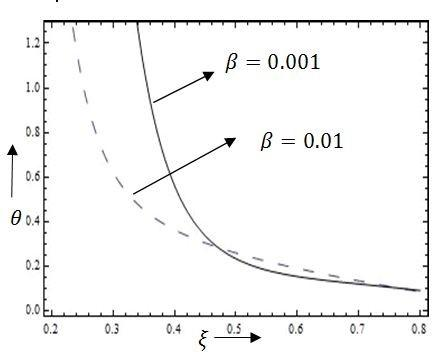Figure 3. Temperature profiles for various values of β ## 4.jpg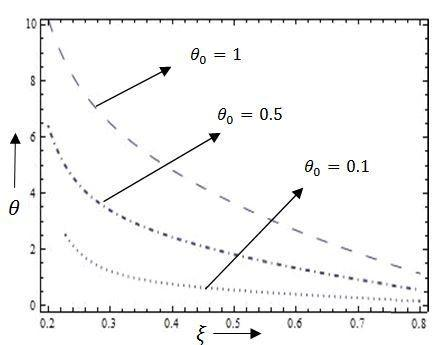Figure 4. Temperature profiles for various values of θ0 The geometry is also affecting factor for temperature of fluid. Figures 5-7 show contour lines for temperature for various area of cross section between concentric ellipses in \xi-\eta plane. As we move from lower vertex (0.2, 0.2) towards opposite vertex of figures, the value of temperature θ decreases. This pattern is visible in all figures. The curves closer to lower vertex of real axis shows magnitude of temperature at inner ellipse, while curves away from real axis are temperature profiles near outer ellipse. As assumed in boundary conditions, all patterns in figures suggest higher temperature at inner surface and lower temperature at outer surface of concentric ellipses. In Figure 5, for \varepsilon=0.28, less contour near outer surface of boundary are visible. This indicates faster diffusion of heat, away from inner surface. This is due to smaller area of cross section created between concentric ellipses at \varepsilon=0.28. From Figures 6, 7 as \varepsilon increases from 0.31 to 0.40, the area of cross section between concentric ellipses also increases. In Figures 6-7, for higher values of \varepsilon, the hotter region more prominent and shows slower rate of heat transfer. This is because increase in \varepsilon, increases the area of cross section and availability of larger area of cross section causes heat transfer to slow down towards outer boundary. Thus more contour lines suggest less temperature at outer ellipse. Thus it is evident that larger area of cross section between concentric ellipses slows down the rate of heat transfer near boundaries. ## 5.jpg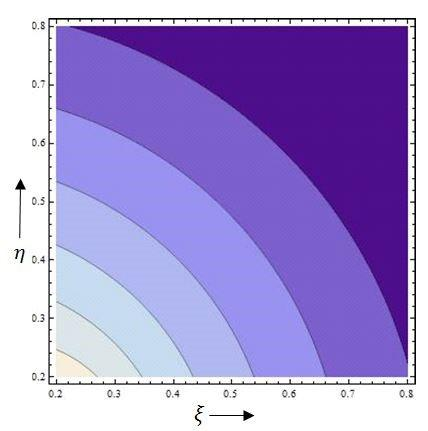Figure 5. Temperature contour profile θ for \varepsilon=0.28 ## 6.jpg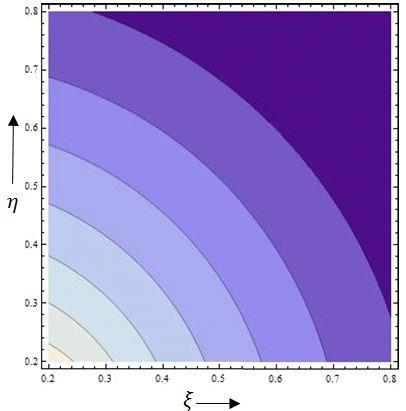Figure 6. Temperature contour profile θ for \varepsilon=0.31 6.3 Velocity profiles The velocity of fluid is analytically evaluated and represented in Eq. (22). The velocity is studied for different values of Grashof number Gr, heat flux β and area of cross section between concentric ellipses \varepsilon=b-a. The velocity profile for G_{r} \rightarrow 0 gives values of velocity in absence of heat transfer. Velocity shows a parabolic profile between two elliptical tubes, which is indicated through rectangular cross section of doubly connected region in \xi-\eta plane. ## 7.jpg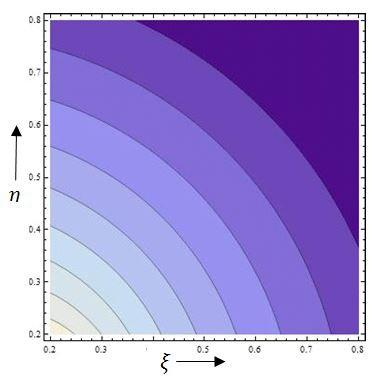Figure 7. Temperature contour profile θ for \varepsilon=0.40 Figure 8 shows the effect of Gr on velocity of fluid for fixed of values of \theta_{0}=0.5, \beta=0.001, \varepsilon=0.31. From Eq. (11) Grashof number Gr is ratio of, product of various parameters such as density \rho, characteristic length h, difference between temperatures of fluid near boundaries, to viscosity of fluid. The variation of density of fluid \rho and viscosity of fluid \mu, caused due to natural convection from inner ellipse to outer ellipse can be seen in variation of Grashof number Gr. Thus the increase in Grashof number Gr, shows the effect of heat transfer on velocity and rate of flow. When Grashof number Gr increases from 1 to 3, the velocity also increases. It can be observed that increase in value of Gr, increases the temperature at wall of inner ellipse, which decreases the viscosity of fluid. Thus the velocity of fluid is enhanced with the greater value of Gr. The same behaviour can be attributed to initial temperature θ0. Figure 9 represents variation of initial temperature θ0 from 0.1 to 1.0 for fixed values of G_{r}=1, \varepsilon=0.31, \beta=0.001. It can be seen from Figure 9 that when initial temperature θ0 is enhanced the velocity of fluid increases. Thus the Grashof number Gr and initial temperature θ0 influences the effect of heat transfer between concentric ellipses on velocity in increasing way. ## 8.jpg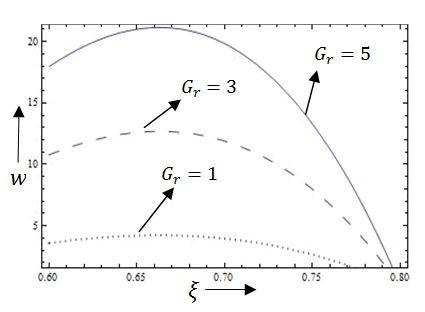Figure 8. Velocity profiles for various values of Gr ## 9.jpgFigure 9. Velocity profiles for various values of θ0 Heat flux β, denotes exchange of thermal energy from fluid to surface of concentric ellipses and vice versa. Figure 10 shows a comparative study of velocity of fluid w for various values of heat flux at \beta=0.01,0.001 with fixed values \varepsilon=0.31, \theta_{0}=0.5, G_{r}=5. When heat flux β increases from 0.001 to 0.01, the velocity decreases. The reduction of rate of heat transfer at the boundaries causes loss in temperature of fluid as observed in section 6.2. Due to decrease in temperature, the viscosity is enhanced which in turn decreases the velocity of fluid. Therefore, contrary to behaviour of Gr and θ0, increase in heat flux β, reduces velocity of fluid flow. ## 10.jpg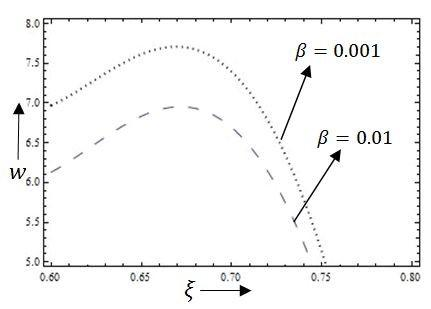Figure 10. Velocity profiles for various values of β Geometry of concentric ellipses also cause change in velocity of fluid flow. Figures 11 to 15 show effect of difference between radii of concentric circles \varepsilon=b-a and thus area of cross section between concentric ellipses, on velocity of fluid. Figure 11 shows effect of smaller area of cross section on velocity at \varepsilon=0.28. Comparing Figure 11 and 12 it can be assessed that when area of cross section is smaller there will be less flow and hence magnitude of velocity will be less. Figure 13 shows velocity at \varepsilon=0.40 which has larger area of cross section and can be seen enhancement in magnitude of velocity. Figure 14 shows comparative study of flow velocity for different area of cross section between concentric ellipses at \varepsilon=0.28, 031, 0.40. Thus it is visible from Figures 11 to 14 that as \varepsilon increases, in presence of heat transfer, the magnitude of velocity will be high. This is due to availability of larger area of cross section for flow. Figure 15 shows variation of velocity of fluid for different \varepsilon=031, 0.40, in the absence of heat transfer (\mathrm{G}_{\mathrm{r}} \rightarrow 0 and \beta=0). In this case also, the velocity enhances with larger area of cross section. Comparing Figures 14-15, the presence of heat transfer improves velocity much compared to velocity in absence of heat transfer. The effect of increase in area of cross section on improving the velocity of flow can be seen here too. ## 11.jpg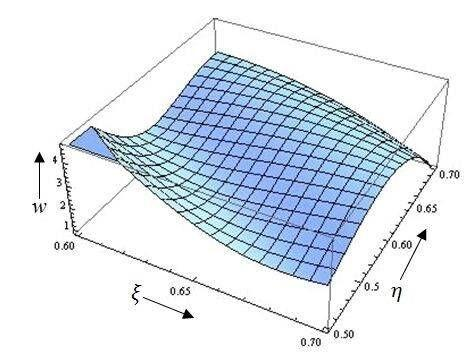Figure 11. Velocity profile w along axial axes (\xi, \eta) for \varepsilon=0.28, \theta_{0}=0.5, \beta=0.001, G_{r}=5 ## 12.jpg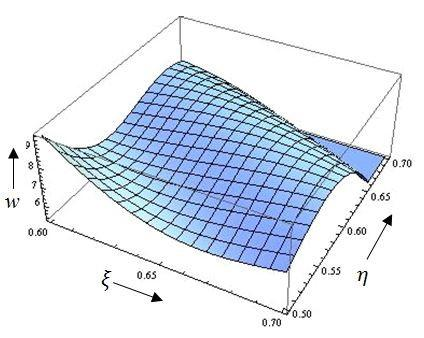Figure 12. Velocity profile w along axial axes (\xi, \eta) for \varepsilon=0.31, \theta_{0}=0.5, \beta=0.001, G_{r}=5 ## 13.jpg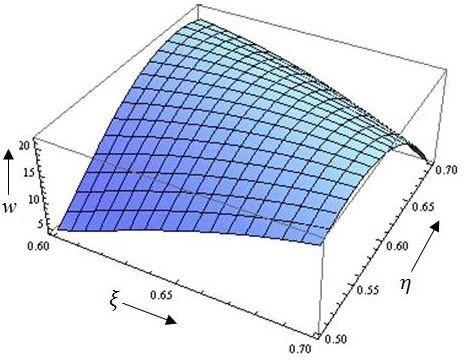Figure 13. Velocity profile w along axial axes (\xi, \eta) for \varepsilon=0.40, \theta_{0}=0.5, \beta=0.001, G_{r}=5 ## 14.jpg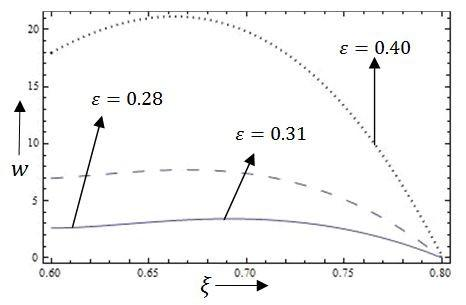Figure 14. Velocity profiles for various values of \varepsilon and for \theta_{0}=0.5, \beta=0.001, G_{r}=5 ## 15.jpg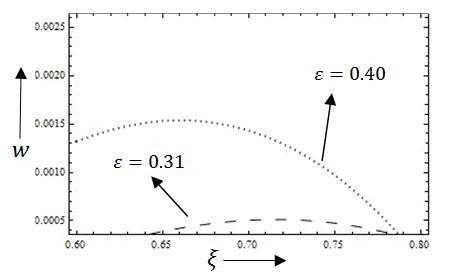Figure 15. Velocity profiles for various values of \varepsilon and for \theta_{0}=0, \beta=0, G_{r}=0 6.3 Rate of flow The rate of flow is computed from Eq. (26) and studied, by varying parameters such as \theta_{0}, G_{r}, \varepsilon which affect the rate of flow. Table 2. Rate of flow computed for various values of \theta_{0}, G_{r}, \varepsilon and at fixed value of β=0.001  1. β 2. θ0 3. Gr ε =0.28 ε=0.31 ε=0.40 0 0 0 0.12745 0.5517 4.95717 0.001 0.1 1 0.12745 0.5517 4.95717 0.5 47.7102 179.002 617.009 1 244.105 940.354 4340.37 0.1 5 489.6 1892.04 8994.58 0.5 238.041 892.804 3065.22 1 1220.02 4699.56 21682.2 0.1 10 2447.49 9458.01 44953.1 0.5 475.955 1785.06 6125.47 1 2439.91 9398.58 43359.1 Table 2 lists the different values of rate of flow for fixed value of β=0.001 and for various values of initial temperature θ0=0.1, 0.5, 1, Grashof number Gr =1, 5, 10 and \varepsilon=0.28,0.31,0.40. A case of rate of flow in the absence of heat transfer is studied by setting parameters \theta_{0}, G_{r}, \beta to zero for various ε. These values are used to compare rate of flow with previous work . In Table 1, when parameter ε increased from 0.28, 0.31, 0.40, there is subsequent increase in rate of flow. This is due to increase in magnitude of velocity with increase in area of cross section of concentric ellipses as studied in section 6.3 Also, when initial temperature θ0 is raised from 0.1, 0.5 to 1 corresponding rate of flow is increased for fixed Gr =1. The same behaviour can be observed in case of Gr . Thus when θ0, Gr and ε increases, corresponding rate of flow also increases. 6.4 Shear stress at wall of elliptical tubes The normal shear stress at inner wall and outer wall of concentric ellipses is given by Eqns. (31) and (32) and same are represented graphically. The shear stress at inner wall \tau_{\text {inner }} and outer wall \tau_{\text {outer }} is calculated along surface \rho=a and \rho=b respectively. The graphs of \tau_{\text {inner }} and \tau_{\text {outer }} are plotted against \xi axis at fixed \eta for various values of Grashof number Gr =1, 1.05 and heat flux β=0.001, 0.002. ## 16.jpgFigure 16. Shear stress at inner wall of concentric ellipses \tau_{\text {inner }} for various values of \beta ## 17.jpg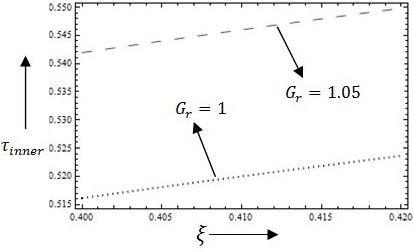Figure 17. Shear stress at inner wall of concentric ellipses \tau_{\text {inner }} for various values of G_{r}. The graphs of \tau_{\text {inner }} and \tau_{\text {outer }} follows the rule of viscosity and proportional to the velocity of fluid flow. Figure 16 represents graph of shear stress at inner wall of concentric ellipses \tau_{\text {inner }} for various values of Gr. It can be seen that for small increase in Gr, shear stress \tau_{\text {inner }} increases considerably. The considerable increase in velocity for small increase in values of Gr is reason for this behaviour of \tau_{\text {inner }}. Figure 17 demonstrates that shear stress \tau_{\text {inner }} decreases with increase in heat flux β. This behaviour follows from decrease in velocity of fluid with increase in β, as seen in section 6.3. ## 18.jpg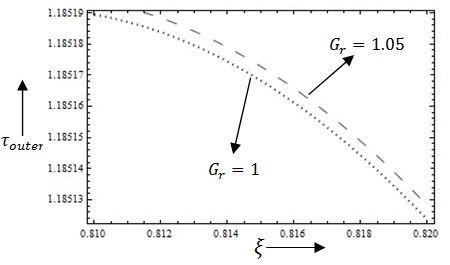Figure 18. Shear stress at outer wall of concentric ellipses \tau_{\text {outer }} for various values of G_{r} ## 19.jpg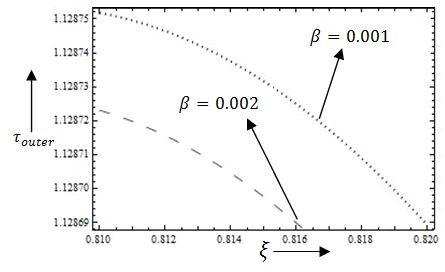Figure 19. Shear stress at outer wall of concentric ellipses \tau_{\text {outer }} for various values of \beta The variation of shear stress at outer wall of concentric ellipses \tau_{\text {outer }} are given by Figures 18 and 19 for various values of G_{r} and \beta . \tau_{\text {outer }} shows decreasing curve due to sticking of fluid particles near outer wall. The Figure 18 shows that shear stress at outer wall \tau_{\text {outer }} is enhanced with small increase in Gr while Figure 19 shows \tau_{\text {outer }} depletes with increase in heat flux β. Thus it can be concluded that shear stress at inner and outer wall of concentric ellipses follows the law of viscosity and is proportional to velocity gradient. 7. Numerical Solution of Velocity in Absence of Heat Transfer and Comparison with Analytical Solution In this section, an attempt is made to compare numerical solution of velocity in absence of heat transfer (G_{r} \rightarrow 0, \beta=0, \theta_{0}=0), by adopting the transformation used in D’Alessio and Dennis  which maps concentric ellipses to rectangular region, and later using finite difference scheme to find velocity. The velocity in Eq. (3) of present study in absence of heat transfer is given by, \frac{\partial^{2} \mathrm{w}}{\partial \mathrm{x}^{2}}+\frac{\partial^{2} \mathrm{w}}{\partial \mathrm{y}^{2}}=\frac{1}{\mu} \frac{\partial \mathrm{p}}{\partial \mathrm{z}} (33) Non- dimensionalizing Eq. (33) using parameters in Eq. (8) and neglecting (*), the Eq. (33) reduces to, \frac{\partial^{2} w}{\partial x^{2}}+\frac{\partial^{2} w}{\partial y^{2}}=\frac{\partial p}{\partial z} (34) Following D’Alessio and Dennis , the concentric ellipse C1 and C2 in Eq. (1) are transformed to rectangular strip using mapping, x+i y=\operatorname{Cosh}(\xi+i(\theta+\mathrm{A})) (35) where, \xi_{1} \leq \xi \leq \xi_{2} and -\pi \leq \theta \leq \pi and \mathrm{A} is inclination angle. The values of \xi are taken along horizontal axis of mesh which are determined following . The values of \xi are given by \tanh \xi_{1}=\frac{\beta_{1}}{\alpha_{1}} and \tanh \xi_{2}=\frac{\beta_{2}}{\alpha_{2}} which specifies the ratio of minor axis to major axis of concentric ellipses. Due to symmetry along y axis, \theta is varied from -\pi to \pi and is considered vertical axis in rectangular mesh. Applying conformal map in Eq. (35) to Eq. (34), the transformed form of Eq. (34) in (\xi, \theta) co-ordinates is given by, \frac{\partial^{2} w}{\partial \xi^{2}}+\frac{\partial^{2} w}{\partial \theta^{2}}=\frac{\partial p}{\partial z}\left(\begin{array}{c}\cosh ^{2}(\xi) \sin ^{2}(\theta+\alpha)+ \\ \sinh ^{2}(\xi) \cos ^{2}(\theta+\alpha)\end{array}\right) (36) Using finite difference scheme of numerical solution to Eq. (36), the solution for velocity w_{i, j} is obtained by varying horizontal strips \xi=\xi_{1} to \xi=\xi_{2} and vertical strips \theta=-\pi to \theta=\pi with \delta_{\xi}=\frac{\xi_{2}-\xi_{1}}{n}, \delta_{\theta}=\frac{2 \pi}{n} as step size, where n is number of intervals mesh to be divided. The boundary values of rectangular mesh is taken by incorporating no- slip boundary condition w=0 at \xi=\xi_{1} to \xi=\xi_{2} and symmetry condition at \theta=-\pi to \pi. Figures 20 and 21 show the graph of both numerical and analytical solution for various area of cross section \varepsilon, in absence of heat transfer. The graphs show similar characteristic of velocity obtained using both numerical and analytical approach with little deviation. Comparing graphs in Figures 20 and 21, we can observe that velocity obtained from both numerical and analytical approach increases with increase in area of cross section of concentric ellipses from ε=0.28 to ε=0.31. Since results from both methods are approximately equal, the computation of velocity using the analytical approach in present study can be extended to inclusion of effect of heat transfer. ## 20.jpgFigure 20. Velocity profiles w along ξ axis for \varepsilon=0.28 in absence of heat transfer G_{r}=0, \beta=0, \theta_{0}=0 ## 21.jpg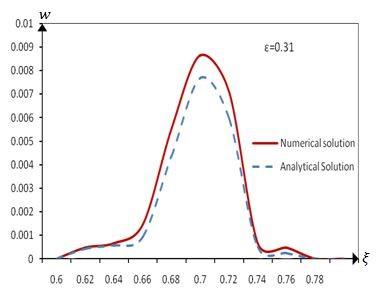Figure 21. Velocity profiles w along ξ axis for \varepsilon=0.31 in absence of heat transfer G_{r}=0, \beta=0, \theta_{0}=0 8. Conclusions The present study effectively analyses the flow of Newtonian fluid between concentric elliptic cylinders kept at different temperatures. The effect of heat transfer on velocity and rate of flow are studied by varying parameters G_{r}, \theta_{0}, \beta and \varepsilon. Ellipse creates a larger area of cross section due to its geometry and hence provides more fluid to pass through. Usage of conformal mapping helps to map region which is not in perfect symmetry to region having perfect symmetry. Thus the results of former region can be studied through later region by mapping. Variation different parameters on the flow is represented graphically which proves that the effect of heat transfer has great influence on velocity and rate of flow. Inclusion of heat transfer through parameter Gr enhances magnitude of velocity and hence rate of flow considerably. Thus, increase in temperature of fluid increases the velocity of fluid and rate of flow and thermal exchange can be maintained with heat flux \beta. Acknowledgement The authors thank the management of Nitte Meenakshi Institute of technology and REVA University for their support in carrying out this research work. Nomenclature  \frac{\mathrm{dp}}{\mathrm{d} z} pressure gradient in normal direction Cv specific heat capacity Gr Grashof Number \overrightarrow{\mathrm{q}}=\mathrm{u}(\mathrm{x}, \mathrm{y}, \mathrm{z}) \hat{\mathrm{\imath}}+$$+\mathrm{v}(\mathrm{x}, \mathrm{y}, \mathrm{z}) \hat{\mathrm{\jmath}}+$$\mathrm{w}(\mathrm{x}, \mathrm{y}, \mathrm{z}) \hat{\mathrm{k}} velocity of the fluid \tilde{s} Stress tensor c1 Inner ellipse c2 Outer ellipse \alpha_{1} major axis of inner ellipse \beta_{1} minor axis of inner ellipse \alpha_{2} major axis of outer ellipse \beta_{2} minor axis of outer ellipse a radius of inner circle after conformal map of ellipse c1 b radius of outer circle after conformal map of ellipse c2 \varepsilon=b-a parameter representing area of cross section w dimensionless velocity in z direction h=\alpha_{1}^{2}-\beta_{1}^{2}=\alpha_{2}^{2}-$$\beta_{2}^{2}$ characteristic length representing difference between diameter of ellipses Greek symbols $\rho$ density of fluid κ Co-efficient of thermal conductivity $\phi$ Viscous dissipation $\alpha$ thermal diffusivity $\theta$ dimensionless temperature of fluid $\beta$ heat flux $\mu$ Coefficient of viscosity of fluid $\theta_{0}$ Initial temperature at wall of inner ellipse $\tau_{\text {inner }}$ Shear stress at inner ellipse $\tau_{\text {outer }}$ Shear stress at outer ellipse
Appendix

$A_{11}=\left(\rho^{2}+\frac{\lambda^{2}}{\rho^{2}}\right)+\frac{\lambda}{\rho^{2}}\left(\zeta^{2}+\frac{\rho^{4}}{\zeta^{2}}\right)$,

$A_{12}=\left(\rho^{2}+\frac{\lambda^{2}}{\rho^{2}}\right)^{2}+\frac{\lambda^{2}}{\rho^{4}}\left(\zeta^{4}+\frac{\rho^{8}}{\zeta^{4}}+2 \rho^{4}\right)+2\left(\rho^{2}+\frac{\lambda^{2}}{\rho^{2}}\right)\left(\zeta^{2}+\frac{\rho^{4}}{\zeta^{2}}\right)$,

\begin{aligned} A_{13}=& \rho^{2}\left(2 B \log \rho+2 b_{0}\right)\left(1+\frac{\lambda^{2}}{\rho^{4}}\right)-\lambda\left(2 B \log \rho+2 b_{0}\right) \\ &\left(\frac{\zeta^{2}}{3}-\frac{\rho^{4}}{\zeta^{2}}\right)+\rho^{2}\left(b_{2}+\frac{b_{-2}}{\rho^{4}}\right)\left(1+\frac{\lambda^{2}}{\rho^{4}}\right)\left(\frac{\zeta^{2}}{3}-\frac{\rho^{4}}{\zeta^{2}}\right)+\\ & \rho^{2}\left(b_{2}+\frac{b_{-2}}{\rho^{4}}\right)\left(1+\frac{\lambda^{2}}{\rho^{4}}\right)\left(\frac{\zeta^{2}}{3}-\frac{\rho^{4}}{\zeta^{2}}\right)-\lambda\left(b_{2}+\frac{b_{-2}}{\rho^{4}}\right)\left(\frac{\zeta^{4}}{5}-\frac{\rho^{8}}{3 \zeta^{4}}\right) \\ &-2 \lambda \rho^{4}\left(b_{2}+\frac{b-2}{\rho^{4}}\right) . \end{aligned},

$A_{14}=\left(\mathrm{b}^{4}-\frac{\lambda^{4}}{\mathrm{~b}^{4}}\right)-\left(\mathrm{a}^{4}-\frac{\lambda^{4}}{\mathrm{a}^{4}}\right), A_{15}=\left(\mathrm{b}^{2}-\frac{\lambda^{2}}{\mathrm{~b}^{2}}\right)-\left(\mathrm{a}^{2}-\frac{\lambda^{2}}{\mathrm{a}^{2}}\right)$,

$A_{15}=\left(2 B \log b+2 b_{0}\right)\left(b^{4}+\frac{4 \lambda^{2} b^{2}}{3}-\frac{\lambda^{4}}{b^{4}}\right)$,

$A_{16}=\left(2 B \log a+2 b_{0}\right)\left(a^{4}+\frac{4 \lambda^{2} a^{2}}{3}-\frac{\lambda^{4}}{a^{4}}\right)$,

$A_{17}=\left(\frac{4 \lambda b^{4}}{3}+\frac{4 \lambda^{3}}{3}+2 \lambda\left(b^{6}-\lambda^{2} b^{2}\right)\right)\left(b_{2}+\frac{b_{-2}}{b^{4}}\right)$,

$A_{18}=\left(\frac{4 \lambda a^{4}}{3}+\frac{4 \lambda^{3}}{3}+2 \lambda\left(a 6^{6}-\lambda^{2} a^{2}\right)\right)\left(b_{2}+\frac{b_{-2}}{a^{4}}\right)$,

$A_{19}=\left(\mathrm{F}\left(\mathrm{b}^{2}+\frac{\lambda^{2}}{\mathrm{~b}^{2}}\right)-\frac{2 \lambda \mathrm{f}_{-2}}{\mathrm{~b}^{2}}+2 \lambda \mathrm{f}_{2} \mathrm{~b}^{2}\right)$,

$A_{20}=\left(\mathrm{F}\left(\mathrm{a}^{2}+\frac{\lambda^{2}}{\mathrm{a}^{2}}\right)-\frac{2 \lambda \mathrm{f}_{-2}}{\mathrm{a}^{2}}+2 \lambda \mathrm{f}_{2} \mathrm{a}^{2}\right)$,

$A_{21}=\left(\rho-\frac{\lambda^{2}}{\rho^{3}}\right)+\lambda\left(\frac{\rho}{\zeta^{2}}-\frac{\zeta^{2}}{\rho^{3}}\right)$,

$A_{22}=\left(\rho-\frac{\lambda^{2}}{\rho^{3}}\right)+\lambda\left(\frac{\rho}{\zeta^{2}}-\frac{\zeta^{2}}{\rho^{3}}\right)$,

$A_{23}=\left(\rho-\frac{\lambda^{2}}{\rho^{3}}\right)\left(\zeta^{2}+\frac{\rho^{4}}{\zeta^{2}}\right)+\frac{\rho^{3}}{\zeta^{2}}\left(\rho^{2}+\frac{\lambda^{2}}{\rho^{2}}\right)$,

$A_{24}=\left(2 B \log \rho+2 b_{0}\right)\left(2 \rho-\frac{2 \lambda^{2}}{\rho^{3}}\right)+\frac{2 B}{\rho}\left(\rho^{2}+\frac{\lambda^{2}}{\rho^{2}}\right)$,

$A_{25}=\lambda\left(2 B \log \rho+2 b_{0}\right)\left(\frac{4 \rho^{3}}{\zeta^{2}}\right)-\frac{2 B \lambda}{\rho}\left(\frac{\zeta^{2}}{3}-\frac{\rho^{4}}{\zeta^{2}}\right)$,

$A_{26}=\left(b_{2}+\frac{b_{-2}}{\rho^{4}}\right)\left(\frac{\zeta^{2}}{3}-\frac{\rho^{4}}{\zeta^{2}}\right)\left(2 \rho-\frac{2 \lambda^{2}}{\rho^{3}}\right)$,

$A_{27}=\left(b_{2}+\frac{b_{-2}}{\rho^{4}}\right)\left(\rho^{2}+\frac{\lambda^{2}}{\rho^{2}}\right), A_{28}=\left(\rho^{2}+\frac{\lambda^{2}}{\rho^{2}}\right)\left(\frac{\zeta^{2}}{3}-\frac{\rho^{4}}{\zeta^{2}}\right)$,

$A_{29}=\left(b_{2}+\frac{b_{-2}}{\rho^{4}}\right)\left(\frac{8 \rho^{7}}{3 \zeta^{4}}\right)$,

$A_{30}=\frac{4 b_{2} \lambda}{\rho^{5}}\left(\frac{\zeta^{4}}{5}-\frac{\rho^{8}}{3 \zeta^{4}}\right)$,

$A_{30}=\frac{4 b_{2} \lambda}{\rho^{5}}\left(\frac{\zeta^{4}}{5}-\frac{\rho^{8}}{3 \zeta^{4}}\right)$,

$A_{31}=\left(f_{2}+\frac{f_{-2}}{\rho^{4}}\right), A_{32}=\left(\zeta^{2}+\frac{\rho^{4}}{\zeta^{2}}\right), A_{33}=\left(f_{4}+\frac{f_{-4}}{\rho^{8}}\right)$,

$A_{34}=\left(\zeta^{4}+\frac{\rho^{8}}{\zeta^{4}}\right)$

References

 Khan, W.A., Culham, J.R., Yovanovich, M. (2005). Fluid flow around and heat transfer from elliptical cylinders: Analytical approach. Journal of Thermo Physics and Heat Transfer, 19(2): 178-185. https://doi.org/10.2514/1.10456

 Bharti, R.P., Sivakumar, P., Chhabra, R.P. (2008). Forced convection heat transfer from an ellipticalcylinder to power-law fluids. International Journal of Heat and Mass Transfer, 51(7): 1838-1853. https://doi.org/10.1016/j.ijheatmasstransfer.2007.06.032

 Shivakumar P.N., Ji, C.X. (1993). Poisson’s equation for doubly connected regions. Canadian Applied Mathematics Quarterly, 1: 555-565. http://www.math.ualberta.ca/ami/CAMQ/pdf_files/vol_1/1_4/1_4e.pdf.

 Williams, J.G., Turney, B.W., Moulton, D.E., Waters, S.L. (2020). Effects of geometry on resistance in elliptical pipe flows. Journal of Fluid Mechanics, 891(A4): 1-39. https://doi.org/10.1017/jfm.2020.121

 Saatdjian, E., Midoux, N. (1994). On the solution of Stokes equations between confocal ellipses. Physics of Fluids, 6(12): 3833-3846. https://doi.org/10.1063/1.868375

 Mota, J.P.B., Esteves, I.A.A.C., Portugal, C.A.M., Esperanca, J.M.S.S., Saatdjian, E. (2000). Natural convection heat transfer in horizontal eccentric elliptic annuli containing saturated porous media. International Journal of Heat and Mass Transfer, 43(24): 4367-4379. https://doi.org/10.1016/S0017-9310(00)00068-5

 D’Alessio, S.J.D., Dennis, S.C.R. (1995). Steady laminar forced convection from an elliptic cylinder. Journal of Engineering Mathematics, 29: 181-193. https://doi.org/10.1007/BF00051742

 Saatdjian, E., Midoux, N., GastouChassaing, M.I., Leprevost, J.C., André, J.C. (1996). Chaotic mixing and heat transfer between confocal ellipses: Experimental and numerical results. Physics of Fluids, 8(3): 677-691. https://doi.org/10.1063/1.868853

 Indira, R., Rashmi, K.R., Jayaprakash, M.C. (2018). An analytical study of pulsatile flow of couple stress fluid in doubly connected region. International Journal of Pure and Applied Mathematics, 120(6): 881-893.

 Shivakumar, P.N. (1973). A viscous flow in pipe whose cross section is doubly connected region. Applied Science Results, 27: 355-365. https://doi.org/10.1007/BF00382498

 Haslam, M., Zamir, M. (1998). Pulsatile flow in tubes of elliptic cross sections. Annals of Biomedical Engineering, 26: 780-787. https://doi.org/10.1114/1.106

 Laura, P.A., Faulstich, A.J. (1968). Unsteady heat conduction in plates of polygonal shape. International Journal of Heat and Mass Transfer, 11(2): 297-303. https://doi.org/10.1016/0017-9310(68)90158-0

 Mahadevappa, M., Rammohan Rao, V., Sastri, V.M.K. (1996). Numerical study of steady laminar fully developed fluid flow and heat transfer in rectangular and elliptical ducts rotating about a parallel axis. International Journal of Heat and Mass Transfer, 39(4): 967-875. https://doi.org/10.1016/0017-9310(95)00069-0

 Nejat, A., Mirzakhalili, E., Aliakbari, A., Fallah Niasar, M.S., Vahidkhah, K. (2012). Non-Newtonian power-law fluid flow and heat transfer computation across a pair of confined elliptical cylinders in the line array. Journal of Non-Newtonian Fluid Mechanics, (171-172): 67-82. https://doi.org/10.1016/j.jnnfm.2012.01.007